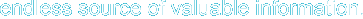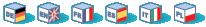# Introduction

The tokenizer functions provide an interface to the PHP tokenizer embedded in the Zend Engine. Using these functions you may write your own PHP source analyzing or modification tools without having to deal with the language specification at the lexical level.

# Requirements

No external libraries are needed to build this extension.

# Installation

Beginning with PHP 4.3.0 these functions are enabled by default. For older versions you have to configure and compile PHP with --enable-tokenizer. You can disable tokenizer support with --disable-tokenizer.

The windows version of PHP has built in support for this extension. You do not need to load any additional extension in order to use these functions.

Note: Builtin support for tokenizer is available with PHP 4.3.0.

# Predefined Constants

The constants below are defined by this extension, and will only be available when the extension has either been compiled into PHP or dynamically loaded at runtime.

T_INCLUDE (integer)

T_INCLUDE_ONCE (integer)

T_EVAL (integer)

T_REQUIRE (integer)

T_REQUIRE_ONCE (integer)

T_LOGICAL_OR (integer)

T_LOGICAL_XOR (integer)

T_LOGICAL_AND (integer)

T_PRINT (integer)

T_PLUS_EQUAL (integer)

T_MINUS_EQUAL (integer)

T_MUL_EQUAL (integer)

T_DIV_EQUAL (integer)

T_CONCAT_EQUAL (integer)

T_MOD_EQUAL (integer)

T_AND_EQUAL (integer)

T_OR_EQUAL (integer)

T_XOR_EQUAL (integer)

T_SL_EQUAL (integer)

T_SR_EQUAL (integer)

T_BOOLEAN_OR (integer)

T_BOOLEAN_AND (integer)

T_IS_EQUAL (integer)

T_IS_NOT_EQUAL (integer)

T_IS_IDENTICAL (integer)

T_IS_NOT_IDENTICAL (integer)

T_IS_SMALLER_OR_EQUAL (integer)

T_IS_GREATER_OR_EQUAL (integer)

T_SL (integer)

T_SR (integer)

T_INC (integer)

T_DEC (integer)

T_INT_CAST (integer)

T_DOUBLE_CAST (integer)

T_STRING_CAST (integer)

T_ARRAY_CAST (integer)

T_OBJECT_CAST (integer)

T_BOOL_CAST (integer)

T_UNSET_CAST (integer)

T_NEW (integer)

T_EXIT (integer)

T_IF (integer)

T_ELSEIF (integer)

T_ELSE (integer)

T_ENDIF (integer)

T_LNUMBER (integer)

T_DNUMBER (integer)

T_STRING (integer)

T_STRING_VARNAME (integer)

T_VARIABLE (integer)

T_NUM_STRING (integer)

T_INLINE_HTML (integer)

T_CHARACTER (integer)

T_ENCAPSED_AND_WHITESPACE (integer)

T_CONSTANT_ENCAPSED_STRING (integer)

T_ECHO (integer)

T_DO (integer)

T_WHILE (integer)

T_ENDWHILE (integer)

T_FOR (integer)

T_ENDFOR (integer)

T_FOREACH (integer)

T_ENDFOREACH (integer)

T_DECLARE (integer)

T_ENDDECLARE (integer)

T_AS (integer)

T_SWITCH (integer)

T_ENDSWITCH (integer)

T_CASE (integer)

T_DEFAULT (integer)

T_BREAK (integer)

T_CONTINUE (integer)

T_OLD_FUNCTION (integer)

T_OLD_FUNCTION is not defined in PHP 5.

T_FUNCTION (integer)

T_CONST (integer)

T_RETURN (integer)

T_USE (integer)

T_GLOBAL (integer)

T_STATIC (integer)

T_VAR (integer)

T_UNSET (integer)

T_ISSET (integer)

T_EMPTY (integer)

T_CLASS (integer)

T_EXTENDS (integer)

T_OBJECT_OPERATOR (integer)

T_DOUBLE_ARROW (integer)

T_LIST (integer)

T_ARRAY (integer)

T_LINE (integer)

T_FILE (integer)

T_COMMENT (integer)

T_ML_COMMENT (integer)

T_ML_COMMENT is not defined in PHP 5. All comments in PHP 5 are of token T_COMMENT.

T_DOC_COMMENT (integer)

T_DOC_COMMENT was introduced in PHP 5.

T_OPEN_TAG (integer)

T_OPEN_TAG_WITH_ECHO (integer)

T_CLOSE_TAG (integer)

T_WHITESPACE (integer)

T_START_HEREDOC (integer)

T_END_HEREDOC (integer)

T_DOLLAR_OPEN_CURLY_BRACES (integer)

T_CURLY_OPEN (integer)

T_PAAMAYIM_NEKUDOTAYIM (integer)

T_DOUBLE_COLON (integer)

T_INTERFACE (integer)

PHP 5 only.

T_IMPLEMENTS (integer)

PHP 5 only.

T_CLASS_C (integer)

PHP 5 only.

T_FUNC_C (integer)

PHP 5 only.

T_METHOD_C (integer)

PHP 5 only.

T_ABSTRACT (integer)

PHP 5 only.

T_CATCH (integer)

PHP 5 only.

T_FINAL (integer)

PHP 5 only.

T_INSTANCEOF (integer)

PHP 5 only.

T_PRIVATE (integer)

PHP 5 only.

T_PROTECTED (integer)

PHP 5 only.

T_PUBLIC (integer)

PHP 5 only.

T_THROW (integer)

PHP 5 only.

T_TRY (integer)

PHP 5 only.

T_CLONE (integer)

PHP 5 only.

# Examples

Here is a simple example PHP scripts using the tokenizer that will read in a PHP file, strip all comments from the source and print the pure code only.

Example 1. Strip comments with the tokenizer

 ``` output "as is"                echo \$text;                break;        }    }}?> ```